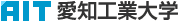日本語
 Please use this identifier to cite or link to this item: `http://hdl.handle.net/11133/2036`

 Title: オイラー法による微分方程式の近似解の誤差評価について Other Titles: On the error estimation of the approximate solution of differential equation by Euler's method Authors: 樋口, 功HIGUCHI, Isao Issue Date: 31-Mar-2003 Publisher: 愛知工業大学 Abstract: By the fundamental theorem of Cauchy-Lipschitz , the existence and the uniqueness of the solution of the following initial value problem are assured: (dy)/(dx)=f(x,y), y(x_0)=y_0 . But we can not obtain the concrete form of the solution by the above fundamental theorem. In some elementary cases, the solution-function can be derived by simple calculations. But in many cases, the solution-function can not be obtained in general. So we need to have their approximate solutions instead of their true solutions with the help of computer. As the methods of finding the approximate solution, the Euler, the Heun and the Runge-Kutta methods are well known. In the present paper, we shall give the error estimation of approximate solution obtained by Euler's method when f(x,y) is Lipschitz continuous. URI: http://hdl.handle.net/11133/2036 Appears in Collections: 38号

Files in This Item:

File Description SizeFormatDSpace Software Copyright © 2002-2010  Duraspace - Feedback